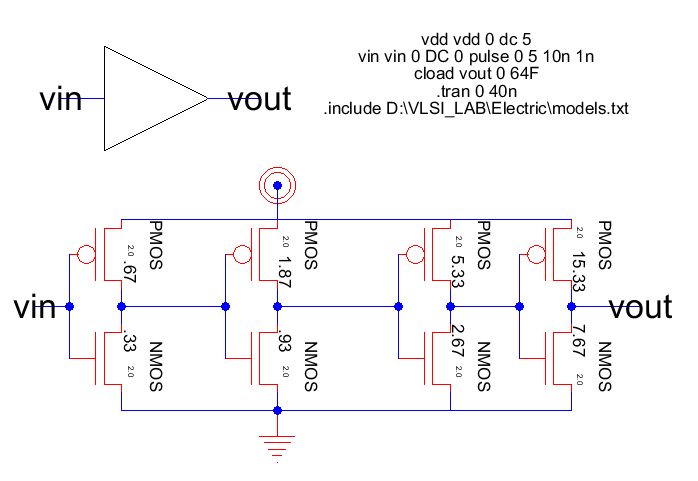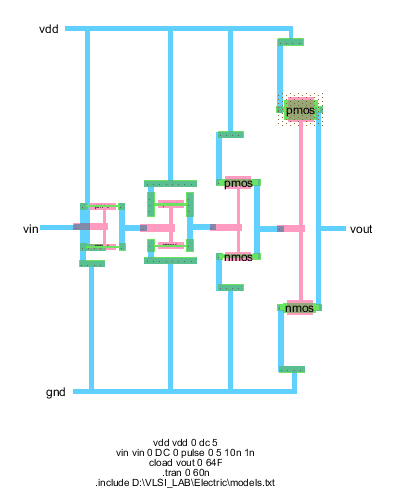## FASTEST BUFFER CIRCUIT

AIM:Design the schematic and layout of the fastest buffer circuit to drive an electrical effort of 64.

CIRCUIT DESIGN Given, electrical effort, H=64
For an inverter logical effort, G=1
Branching effort, B=1 (only one branch exists)
Path effort, F= GBH = 64
Best Number of stages, N=log4 64=3
To get non-inverted output take N=4
Best stage effort, f= F1/N =2.83
Cin i= (Couti x gi)/f
Cin4 = (64X1)/2.83 =>22.6
Wp4 =15.06
Wn4 =7.53
Cin3 = (22.6X1)/2.83 =>7.99
Wp3 =5.32
Wn3 =2.66
Cin4 = (7.99X1)/2.83 =>2.82
Wp2 =1.88
Wn2 =0.94
Cin1 = (2.82X1)/2.83 =>1
Wp1 = 0.67
Wn1 = 0.33
SCHEMATICLAYOUT